Скачать презентацию System Issues Constructing a Computer Algebra System Lecture

4ef669458de201f17881ed199107bfbe.ppt

• Количество слайдов: 46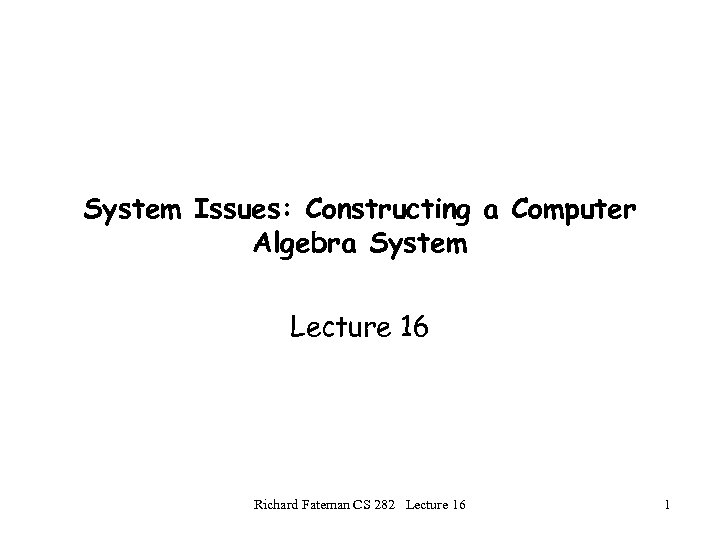System Issues: Constructing a Computer Algebra System Lecture 16 Richard Fateman CS 282 Lecture 16 1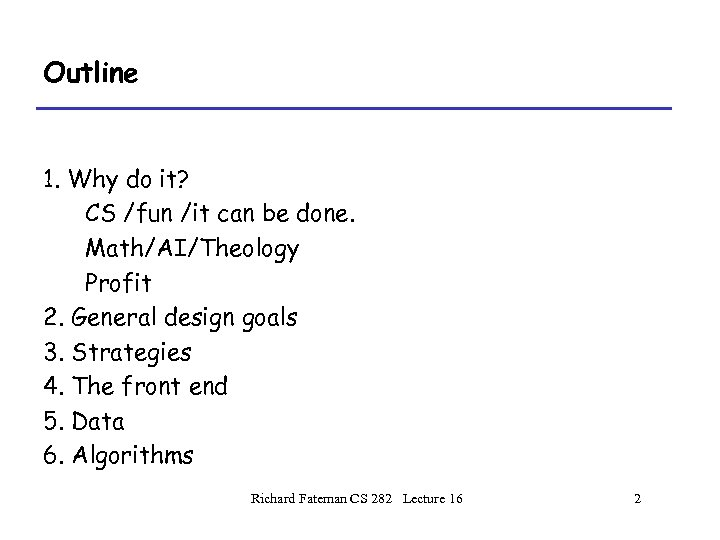Outline 1. Why do it? CS /fun /it can be done. Math/AI/Theology Profit 2. General design goals 3. Strategies 4. The front end 5. Data 6. Algorithms Richard Fateman CS 282 Lecture 16 2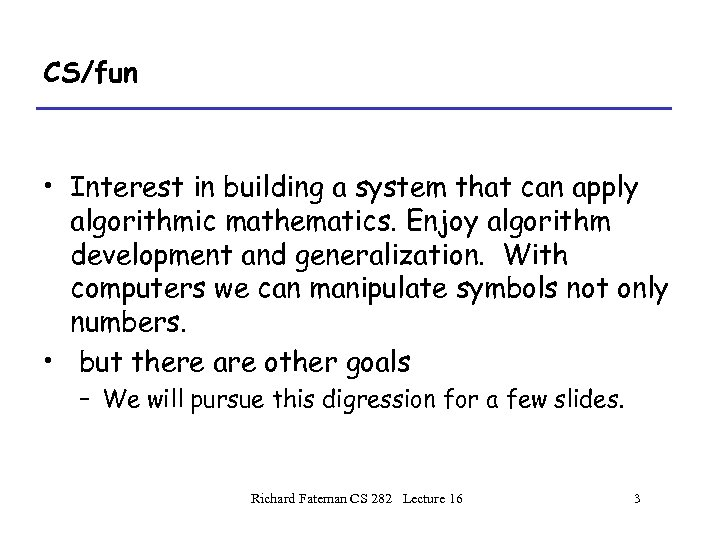CS/fun • Interest in building a system that can apply algorithmic mathematics. Enjoy algorithm development and generalization. With computers we can manipulate symbols not only numbers. • but there are other goals – We will pursue this digression for a few slides. Richard Fateman CS 282 Lecture 16 3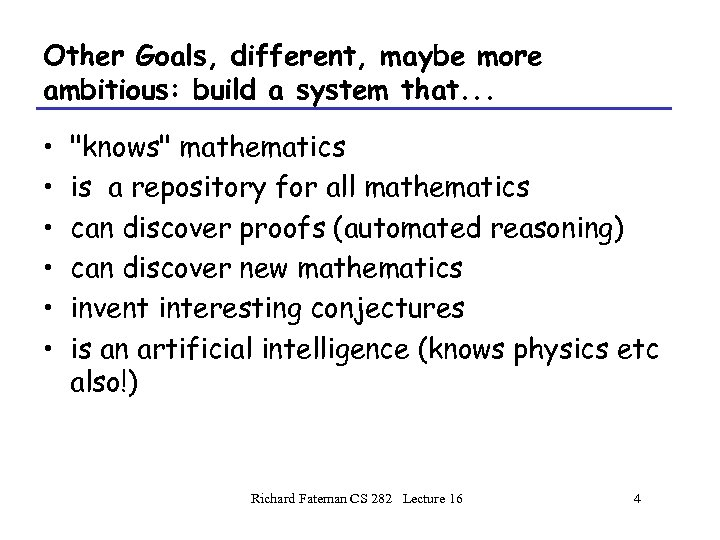Other Goals, different, maybe more ambitious: build a system that. . . • • • "knows" mathematics is a repository for all mathematics can discover proofs (automated reasoning) can discover new mathematics invent interesting conjectures is an artificial intelligence (knows physics etc also!) Richard Fateman CS 282 Lecture 16 4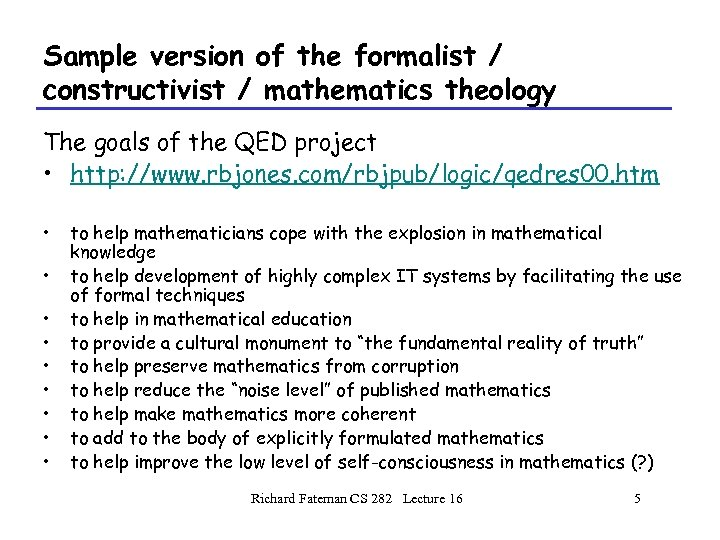Sample version of the formalist / constructivist / mathematics theology The goals of the QED project • http: //www. rbjones. com/rbjpub/logic/qedres 00. htm • • • to help mathematicians cope with the explosion in mathematical knowledge to help development of highly complex IT systems by facilitating the use of formal techniques to help in mathematical education to provide a cultural monument to “the fundamental reality of truth” to help preserve mathematics from corruption to help reduce the “noise level” of published mathematics to help make mathematics more coherent to add to the body of explicitly formulated mathematics to help improve the low level of self-consciousness in mathematics (? ) Richard Fateman CS 282 Lecture 16 5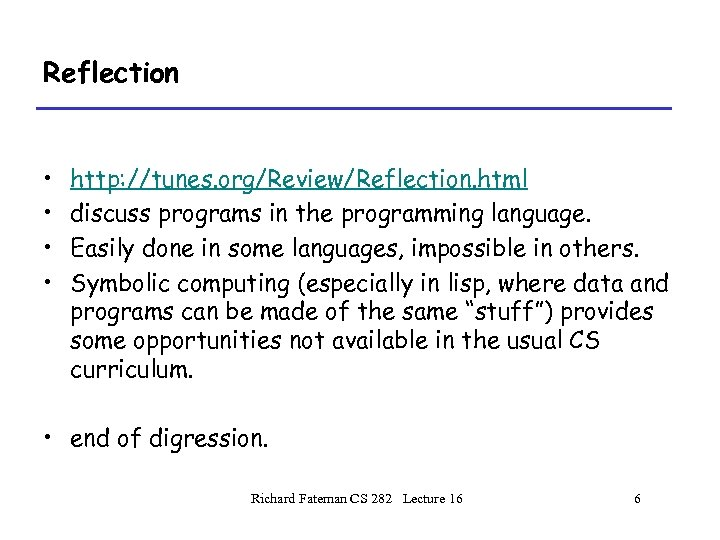Reflection • • http: //tunes. org/Review/Reflection. html discuss programs in the programming language. Easily done in some languages, impossible in others. Symbolic computing (especially in lisp, where data and programs can be made of the same “stuff”) provides some opportunities not available in the usual CS curriculum. • end of digression. Richard Fateman CS 282 Lecture 16 6Can you get rich building a CAS? • What is the market? – – – Fans of higher math? Engineers, Scientists? Wall St. Analysts? Freshman Calculus students? Dentists? (no. ) • How many people will pay how much for this facility? • Find a “killer app”. Probably education. • Find people to gush about it (Steve Jobs? NYTimes? ) Richard Fateman CS 282 Lecture 16 7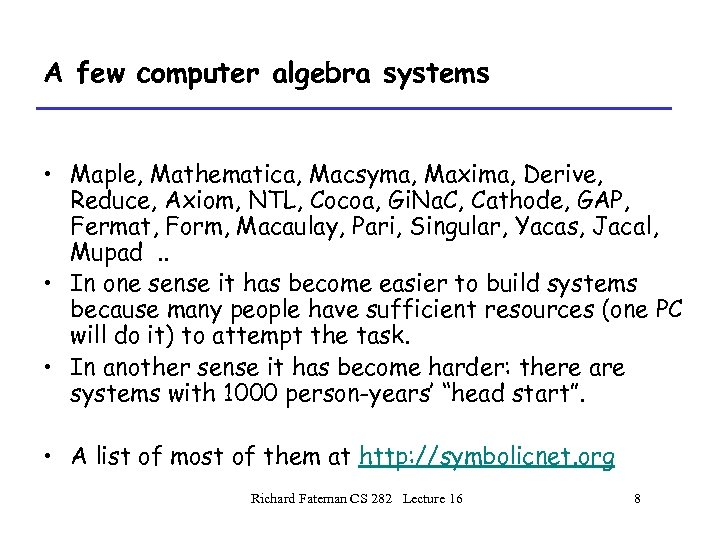A few computer algebra systems • Maple, Mathematica, Macsyma, Maxima, Derive, Reduce, Axiom, NTL, Cocoa, Gi. Na. C, Cathode, GAP, Fermat, Form, Macaulay, Pari, Singular, Yacas, Jacal, Mupad. . • In one sense it has become easier to build systems because many people have sufficient resources (one PC will do it) to attempt the task. • In another sense it has become harder: there are systems with 1000 person-years’ “head start”. • A list of most of them at http: //symbolicnet. org Richard Fateman CS 282 Lecture 16 8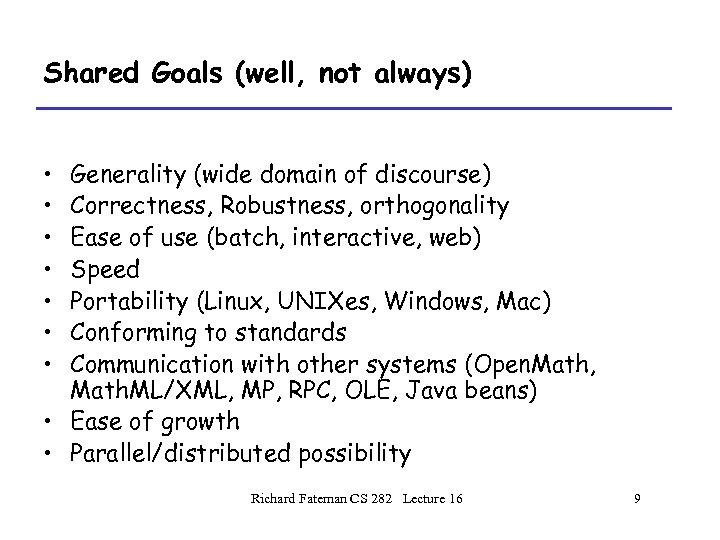Shared Goals (well, not always) • • Generality (wide domain of discourse) Correctness, Robustness, orthogonality Ease of use (batch, interactive, web) Speed Portability (Linux, UNIXes, Windows, Mac) Conforming to standards Communication with other systems (Open. Math, Math. ML/XML, MP, RPC, OLE, Java beans) • Ease of growth • Parallel/distributed possibility Richard Fateman CS 282 Lecture 16 9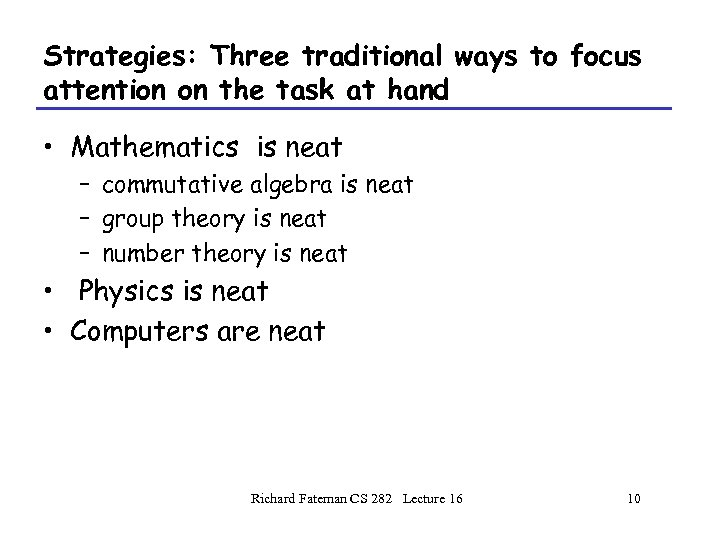Strategies: Three traditional ways to focus attention on the task at hand • Mathematics is neat – commutative algebra is neat – group theory is neat – number theory is neat • Physics is neat • Computers are neat Richard Fateman CS 282 Lecture 16 10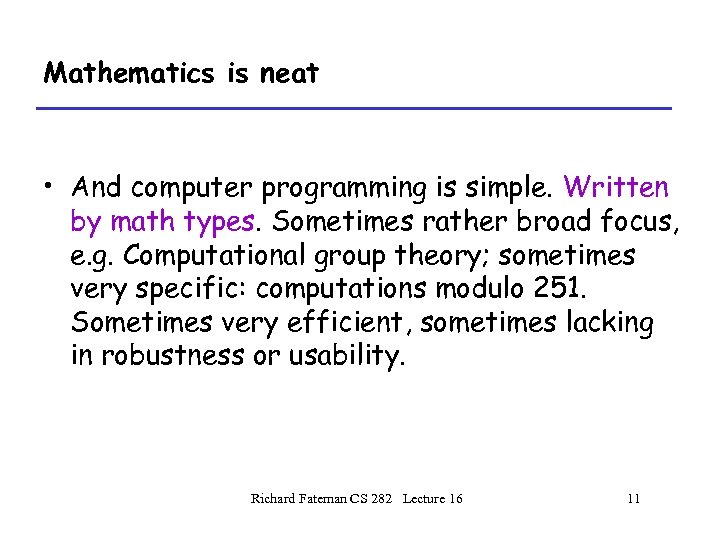Mathematics is neat • And computer programming is simple. Written by math types. Sometimes rather broad focus, e. g. Computational group theory; sometimes very specific: computations modulo 251. Sometimes very efficient, sometimes lacking in robustness or usability. Richard Fateman CS 282 Lecture 16 11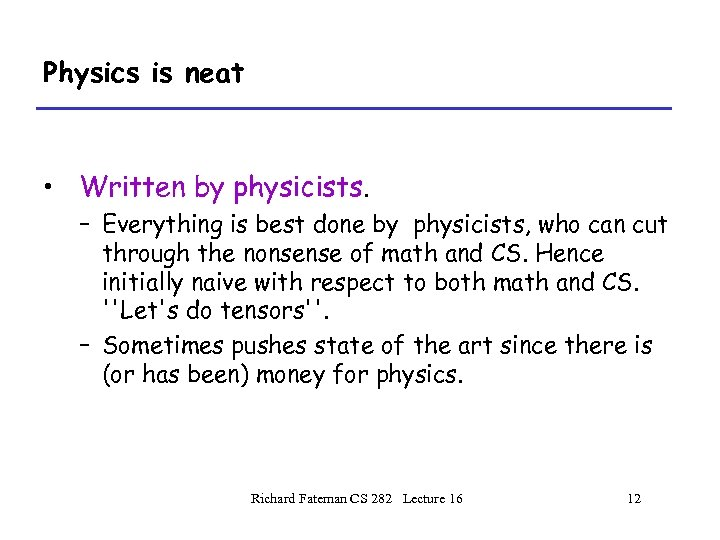Physics is neat • Written by physicists. – Everything is best done by physicists, who can cut through the nonsense of math and CS. Hence initially naive with respect to both math and CS. ''Let's do tensors''. – Sometimes pushes state of the art since there is (or has been) money for physics. Richard Fateman CS 282 Lecture 16 12Computers are neat, math is simple. • Written by CS types. Treat math as a programming language problem, or a data structure problem. • Often mathematically unsophisticated. Sometimes grows in mathematical maturity as CS types perceive gaps and re-engineer. Richard Fateman CS 282 Lecture 16 13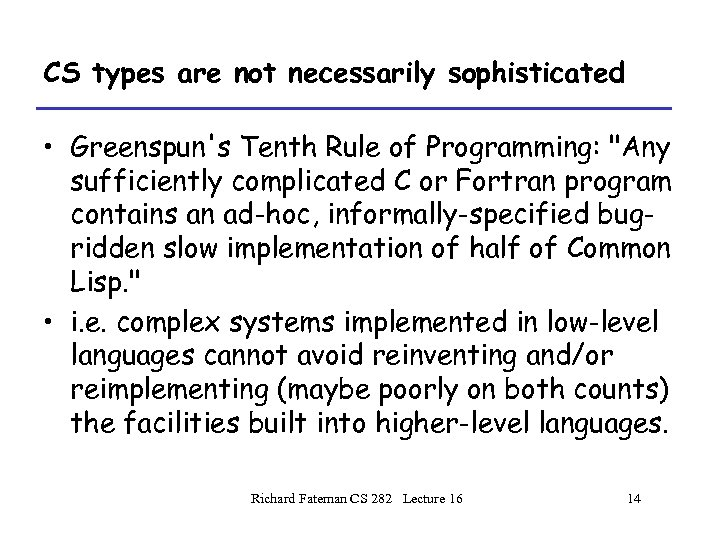CS types are not necessarily sophisticated • Greenspun's Tenth Rule of Programming: "Any sufficiently complicated C or Fortran program contains an ad-hoc, informally-specified bugridden slow implementation of half of Common Lisp. " • i. e. complex systems implemented in low-level languages cannot avoid reinventing and/or reimplementing (maybe poorly on both counts) the facilities built into higher-level languages. Richard Fateman CS 282 Lecture 16 14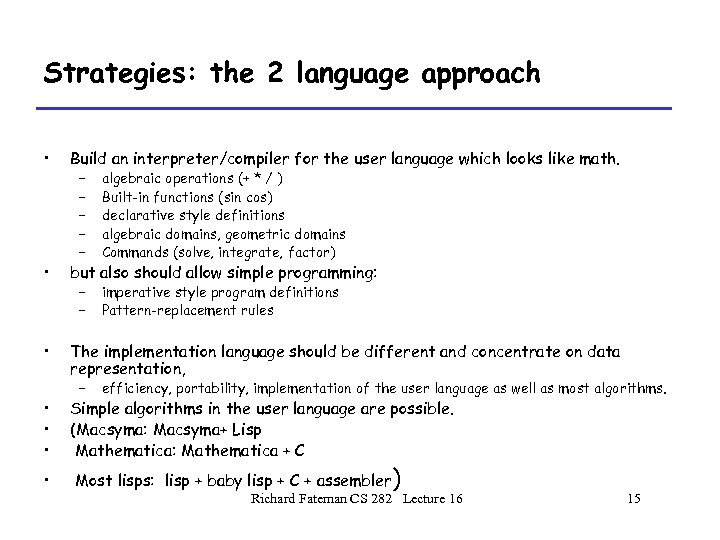Strategies: the 2 language approach • Build an interpreter/compiler for the user language which looks like math. • but also should allow simple programming: • The implementation language should be different and concentrate on data representation, • • – – – algebraic operations (+ * / ) Built-in functions (sin cos) declarative style definitions algebraic domains, geometric domains Commands (solve, integrate, factor) – – imperative style program definitions Pattern-replacement rules – efficiency, portability, implementation of the user language as well as most algorithms. Simple algorithms in the user language are possible. (Macsyma: Macsyma+ Lisp Mathematica: Mathematica + C Most lisps: lisp + baby lisp + C + assembler ) Richard Fateman CS 282 Lecture 16 15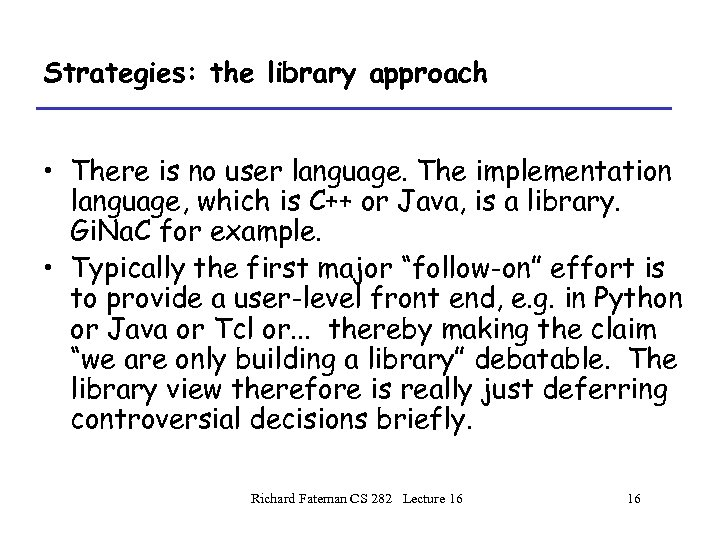Strategies: the library approach • There is no user language. The implementation language, which is C++ or Java, is a library. Gi. Na. C for example. • Typically the first major “follow-on” effort is to provide a user-level front end, e. g. in Python or Java or Tcl or. . . thereby making the claim “we are only building a library” debatable. The library view therefore is really just deferring controversial decisions briefly. Richard Fateman CS 282 Lecture 16 16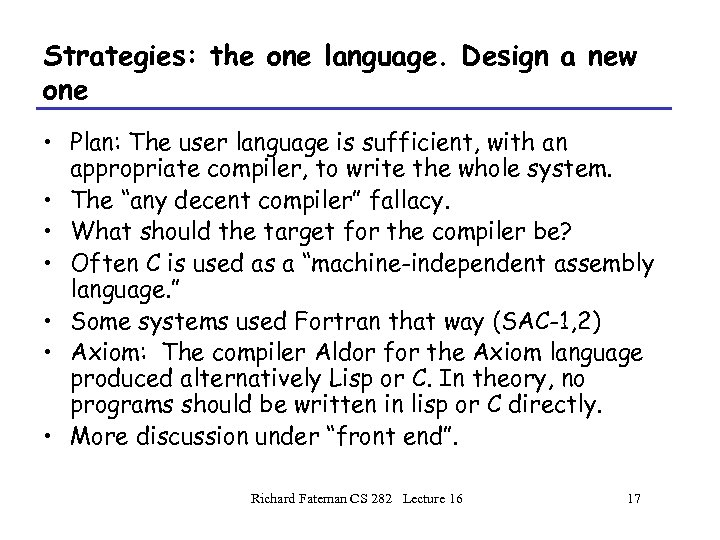Strategies: the one language. Design a new one • Plan: The user language is sufficient, with an appropriate compiler, to write the whole system. • The “any decent compiler” fallacy. • What should the target for the compiler be? • Often C is used as a “machine-independent assembly language. ” • Some systems used Fortran that way (SAC-1, 2) • Axiom: The compiler Aldor for the Axiom language produced alternatively Lisp or C. In theory, no programs should be written in lisp or C directly. • More discussion under “front end”. Richard Fateman CS 282 Lecture 16 17Implementation Language design issues A person or team who invents a language must consider the costs of maintaining that implementation. In at least a few prominent cases, the inventor has little or no experience in languages and makes a muddle of it. Understanding SICP (Abelson/Sussman) would help, in my view. Examples: Macsyma (but that was 1968!), Mathematica, Maple. Even Matlab. Richard Fateman CS 282 Lecture 16 18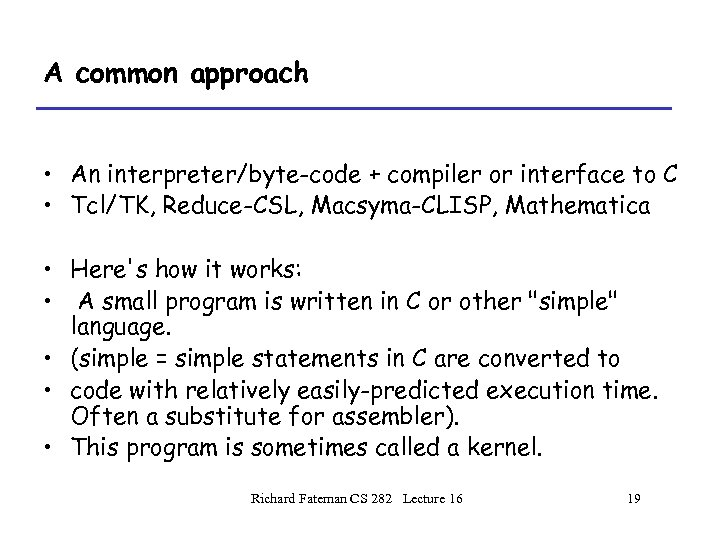A common approach • An interpreter/byte-code + compiler or interface to C • Tcl/TK, Reduce-CSL, Macsyma-CLISP, Mathematica • Here's how it works: • A small program is written in C or other "simple" language. • (simple = simple statements in C are converted to • code with relatively easily-predicted execution time. Often a substitute for assembler). • This program is sometimes called a kernel. Richard Fateman CS 282 Lecture 16 19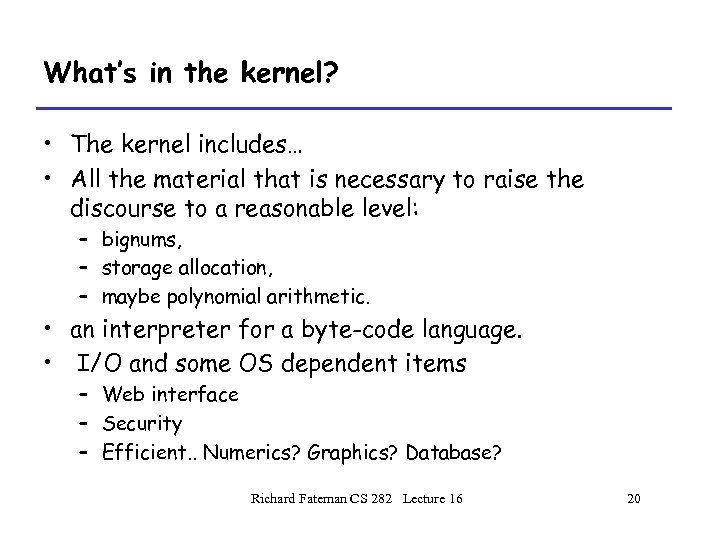What’s in the kernel? • The kernel includes… • All the material that is necessary to raise the discourse to a reasonable level: – bignums, – storage allocation, – maybe polynomial arithmetic. • an interpreter for a byte-code language. • I/O and some OS dependent items – Web interface – Security – Efficient. . Numerics? Graphics? Database? Richard Fateman CS 282 Lecture 16 20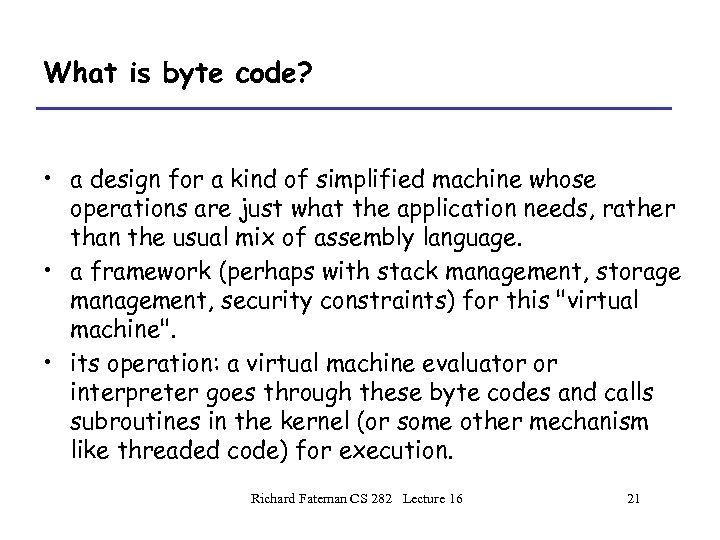What is byte code? • a design for a kind of simplified machine whose operations are just what the application needs, rather than the usual mix of assembly language. • a framework (perhaps with stack management, storage management, security constraints) for this "virtual machine". • its operation: a virtual machine evaluator or interpreter goes through these byte codes and calls subroutines in the kernel (or some other mechanism like threaded code) for execution. Richard Fateman CS 282 Lecture 16 21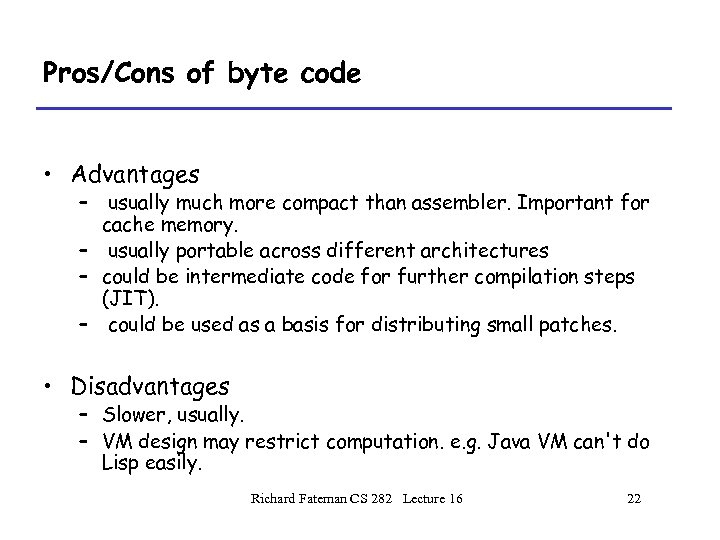Pros/Cons of byte code • Advantages – usually much more compact than assembler. Important for cache memory. – usually portable across different architectures – could be intermediate code for further compilation steps (JIT). – could be used as a basis for distributing small patches. • Disadvantages – Slower, usually. – VM design may restrict computation. e. g. Java VM can't do Lisp easily. Richard Fateman CS 282 Lecture 16 22Mixing byte code and ordinary binary code • CSL approach: (Codemist Standard Language) – While Optimize. More do • {Load the whole system and run for a while as byte-code, profiling. • Compile and reload the parts that seem to be bottlenecks} – Used for implementing Reduce with a modest size memory. – Same data structures • CLISP (implementation of Common Lisp) Richard Fateman CS 282 Lecture 16 23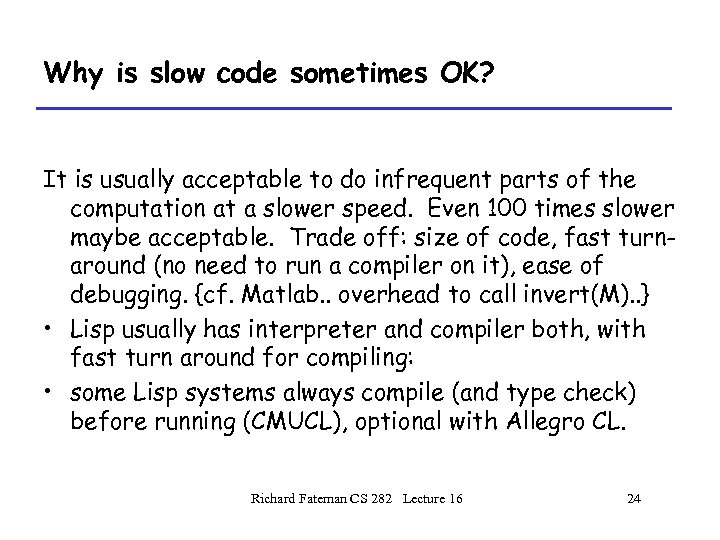Why is slow code sometimes OK? It is usually acceptable to do infrequent parts of the computation at a slower speed. Even 100 times slower maybe acceptable. Trade off: size of code, fast turnaround (no need to run a compiler on it), ease of debugging. {cf. Matlab. . overhead to call invert(M). . } • Lisp usually has interpreter and compiler both, with fast turn around for compiling: • some Lisp systems always compile (and type check) before running (CMUCL), optional with Allegro CL. Richard Fateman CS 282 Lecture 16 24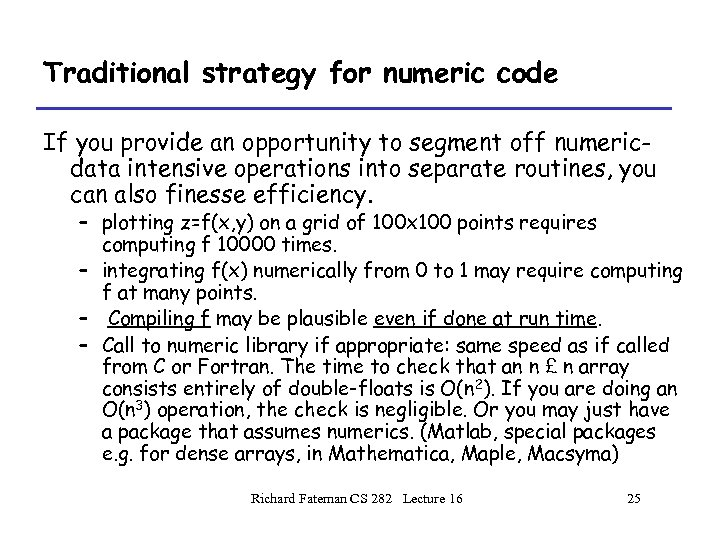Traditional strategy for numeric code If you provide an opportunity to segment off numericdata intensive operations into separate routines, you can also finesse efficiency. – plotting z=f(x, y) on a grid of 100 x 100 points requires computing f 10000 times. – integrating f(x) numerically from 0 to 1 may require computing f at many points. – Compiling f may be plausible even if done at run time. – Call to numeric library if appropriate: same speed as if called from C or Fortran. The time to check that an n £ n array consists entirely of double-floats is O(n 2). If you are doing an O(n 3) operation, the check is negligible. Or you may just have a package that assumes numerics. (Matlab, special packages e. g. for dense arrays, in Mathematica, Maple, Macsyma) Richard Fateman CS 282 Lecture 16 25digression on Reduce • Reduce is written in a dialect called RLISP, which is an infix kind of lisp. e. g. this makes syntactic sense: • a : = list(1, 2, 3); (setq a (list 1 2 3)) • car a + car b ; (+ (car a)(car b)) • There are two modes, algebraic and symbolic (not the clearest names. . . ) • RLISP is implementable in common lisp, CSL, PSL, Scheme. . . Richard Fateman CS 282 Lecture 16 26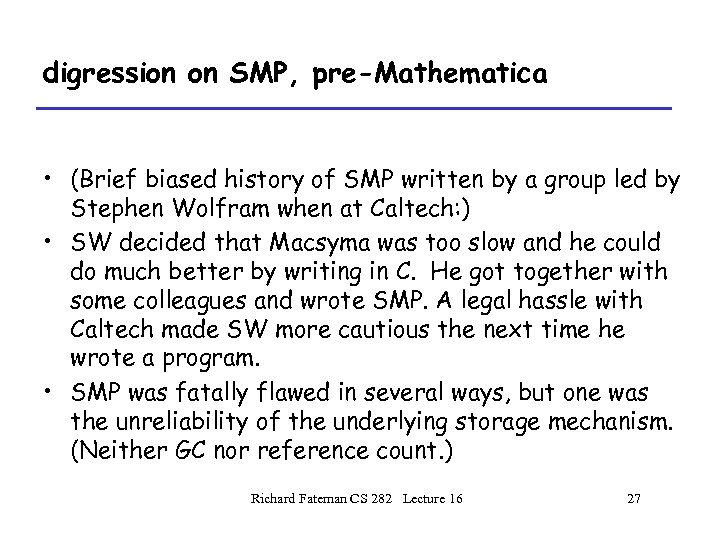digression on SMP, pre-Mathematica • (Brief biased history of SMP written by a group led by Stephen Wolfram when at Caltech: ) • SW decided that Macsyma was too slow and he could do much better by writing in C. He got together with some colleagues and wrote SMP. A legal hassle with Caltech made SW more cautious the next time he wrote a program. • SMP was fatally flawed in several ways, but one was the unreliability of the underlying storage mechanism. (Neither GC nor reference count. ) Richard Fateman CS 282 Lecture 16 27digression on Mathematica • Redid everything to produce Mathematica, which consists of some code written in a customized version of C (actually may be something like Objective C, with some kind of automatic reference counting. ) and the user-language for Mathematica. For Version 4, the code for the kernel consists of about 650, 000 lines of C and 30, 000 lines of Mathematica. • In the Mathematica 4 kernel the breakdown of different parts of the code is roughly as follows: – – language and system: 30%; numerical computation: 25%; algebraic computation: 25%; graphics and kernel output: 20%. • Stats for version 5. 2? Presumably much larger. Richard Fateman CS 282 Lecture 16 28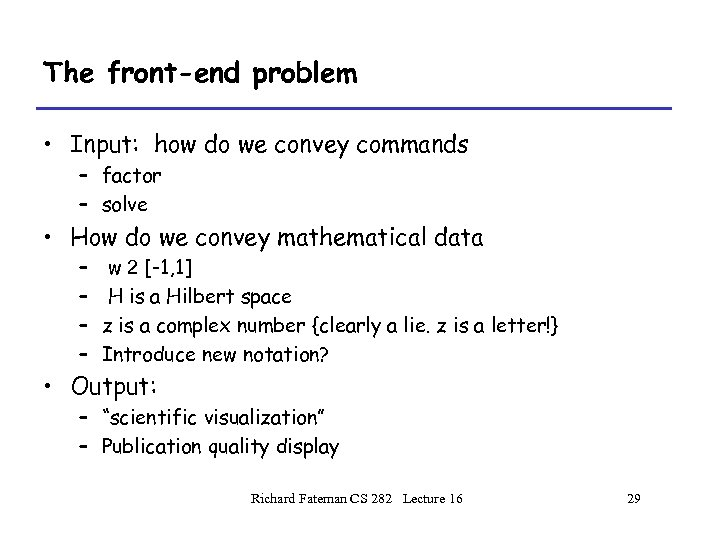The front-end problem • Input: how do we convey commands – factor – solve • How do we convey mathematical data – – w 2 [-1, 1] H is a Hilbert space z is a complex number {clearly a lie. z is a letter!} Introduce new notation? • Output: – “scientific visualization” – Publication quality display Richard Fateman CS 282 Lecture 16 29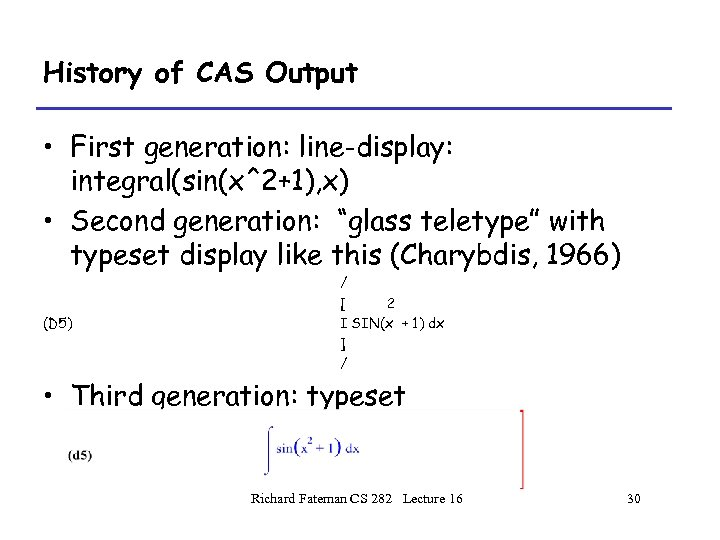History of CAS Output • First generation: line-display: integral(sin(x^2+1), x) • Second generation: “glass teletype” with typeset display like this (Charybdis, 1966) (D 5) / [ 2 I SIN(x + 1) dx ] / • Third generation: typeset Richard Fateman CS 282 Lecture 16 30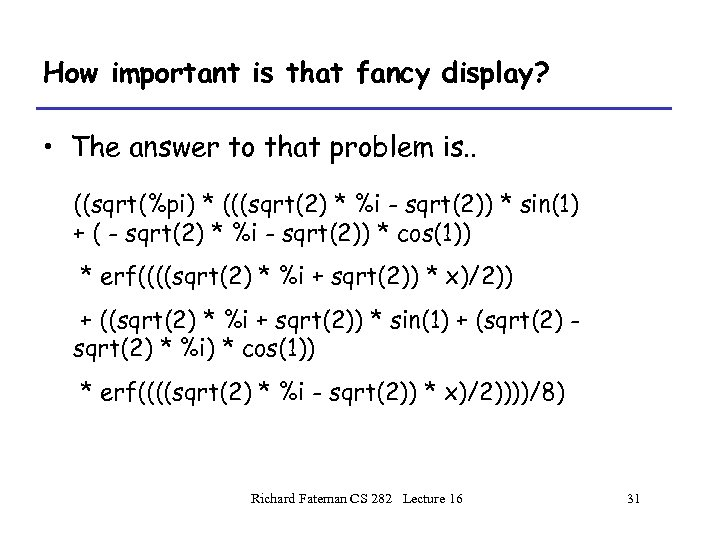How important is that fancy display? • The answer to that problem is. . ((sqrt(%pi) * (((sqrt(2) * %i - sqrt(2)) * sin(1) + ( - sqrt(2) * %i - sqrt(2)) * cos(1)) * erf((((sqrt(2) * %i + sqrt(2)) * x)/2)) + ((sqrt(2) * %i + sqrt(2)) * sin(1) + (sqrt(2) * %i) * cos(1)) * erf((((sqrt(2) * %i - sqrt(2)) * x)/2))))/8) Richard Fateman CS 282 Lecture 16 31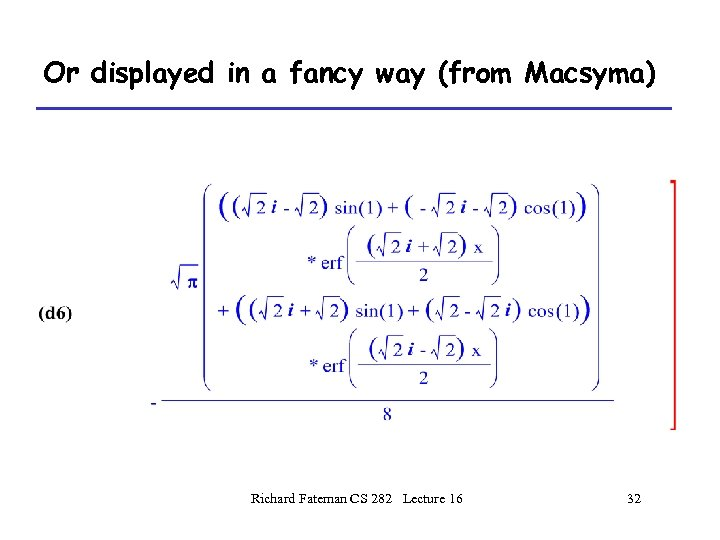Or displayed in a fancy way (from Macsyma) Richard Fateman CS 282 Lecture 16 32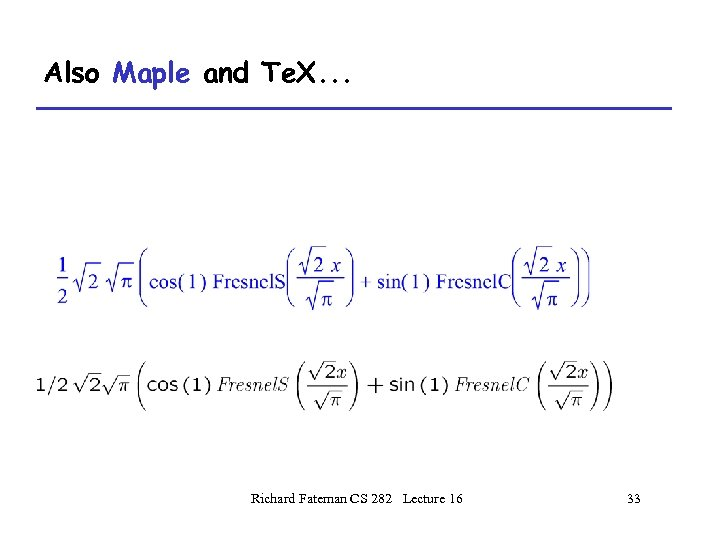Also Maple and Te. X. . . Richard Fateman CS 282 Lecture 16 33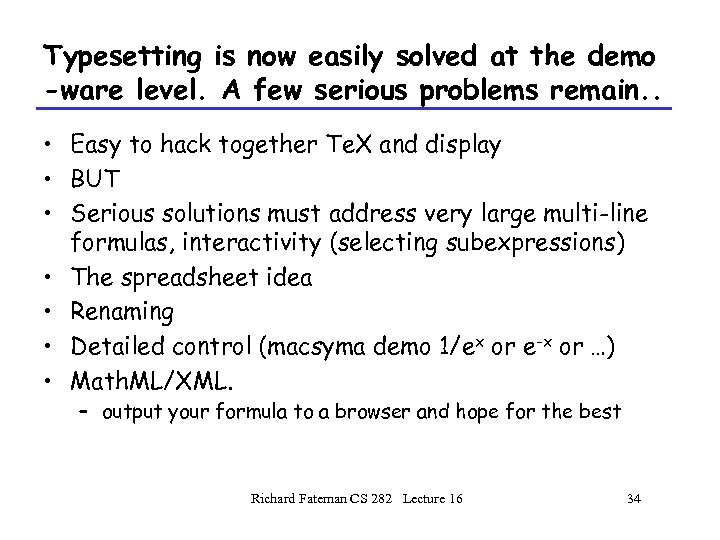Typesetting is now easily solved at the demo -ware level. A few serious problems remain. . • Easy to hack together Te. X and display • BUT • Serious solutions must address very large multi-line formulas, interactivity (selecting subexpressions) • The spreadsheet idea • Renaming • Detailed control (macsyma demo 1/ex or e-x or …) • Math. ML/XML. – output your formula to a browser and hope for the best Richard Fateman CS 282 Lecture 16 34

Our example in Math. ML (generated by Maple) "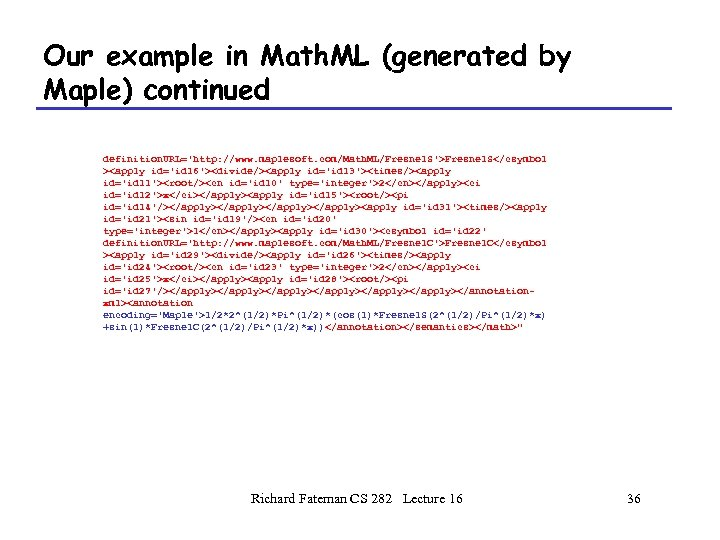Our example in Math. ML (generated by Maple) continued definition. URL='http: //www. maplesoft. com/Math. ML/Fresnel. S'>Fresnel. S2x1Fresnel. C2x1/2*2^(1/2)*Pi^(1/2)*(cos(1)*Fresnel. S(2^(1/2)/Pi^(1/2)*x) +sin(1)*Fresnel. C(2^(1/2)/Pi^(1/2)*x))" Richard Fateman CS 282 Lecture 16 36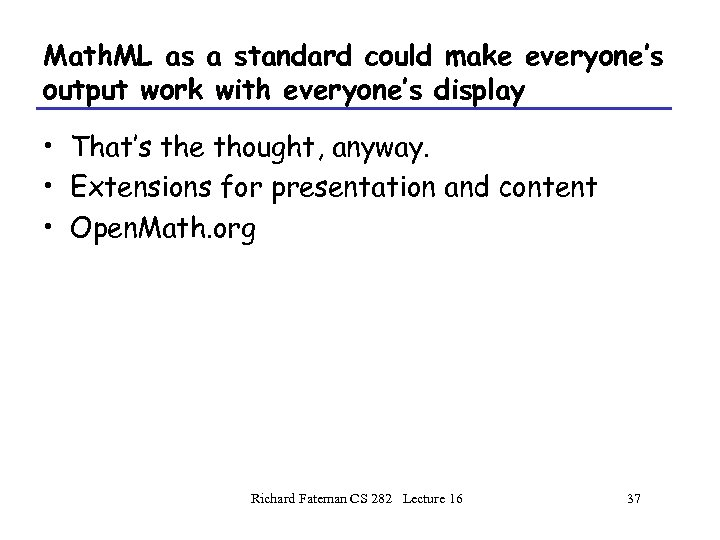Math. ML as a standard could make everyone’s output work with everyone’s display • That’s the thought, anyway. • Extensions for presentation and content • Open. Math. org Richard Fateman CS 282 Lecture 16 37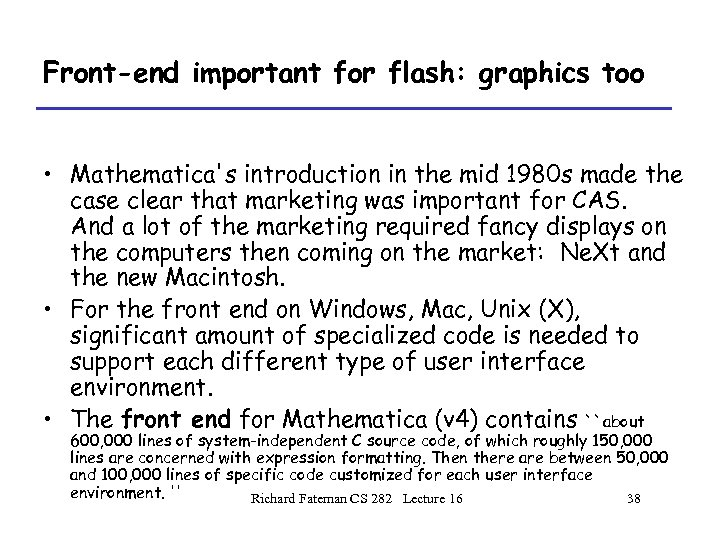Front-end important for flash: graphics too • Mathematica's introduction in the mid 1980 s made the case clear that marketing was important for CAS. And a lot of the marketing required fancy displays on the computers then coming on the market: Ne. Xt and the new Macintosh. • For the front end on Windows, Mac, Unix (X), significant amount of specialized code is needed to support each different type of user interface environment. • The front end for Mathematica (v 4) contains ``about 600, 000 lines of system-independent C source code, of which roughly 150, 000 lines are concerned with expression formatting. Then there are between 50, 000 and 100, 000 lines of specific code customized for each user interface environment. '' Richard Fateman CS 282 Lecture 16 38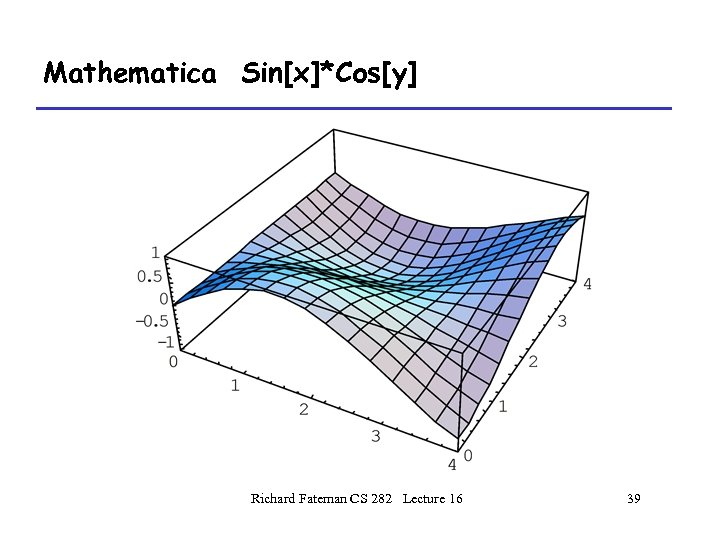Mathematica Sin[x]*Cos[y] Richard Fateman CS 282 Lecture 16 39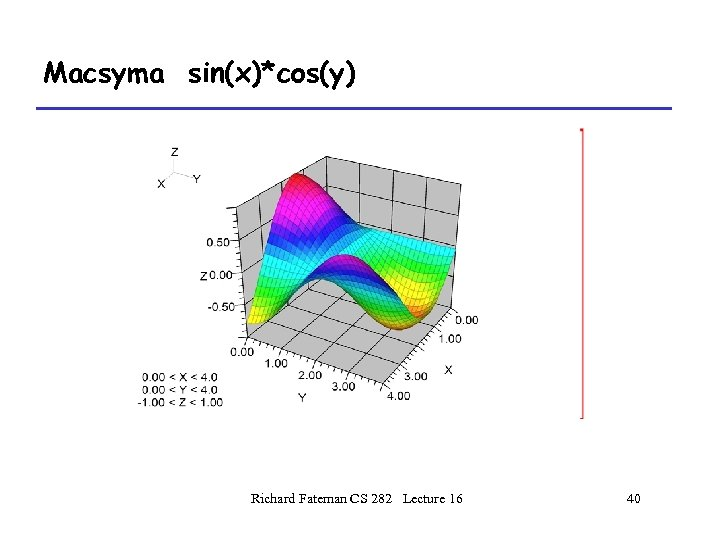Macsyma sin(x)*cos(y) Richard Fateman CS 282 Lecture 16 40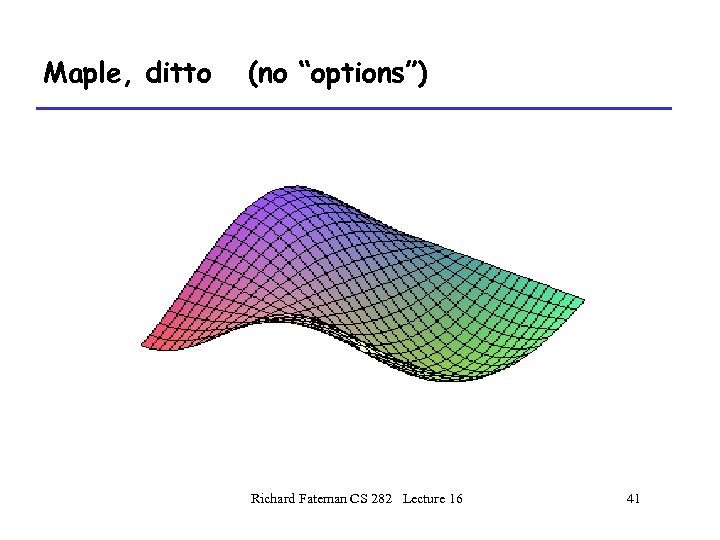Maple, ditto (no “options”) Richard Fateman CS 282 Lecture 16 41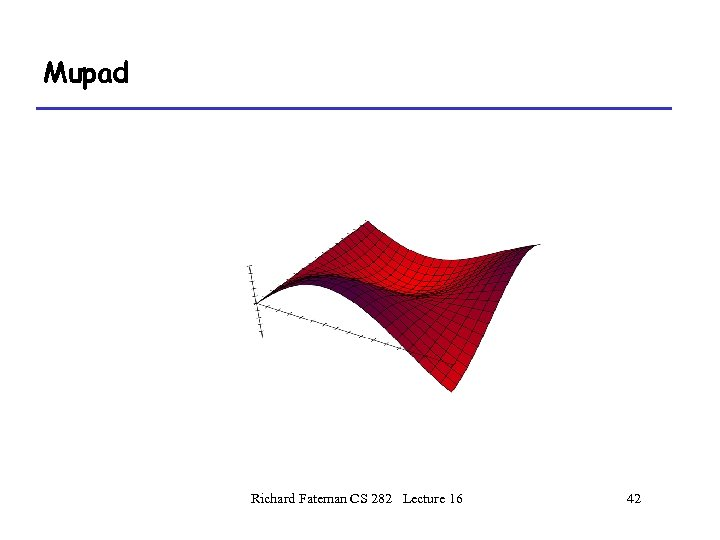Mupad Richard Fateman CS 282 Lecture 16 42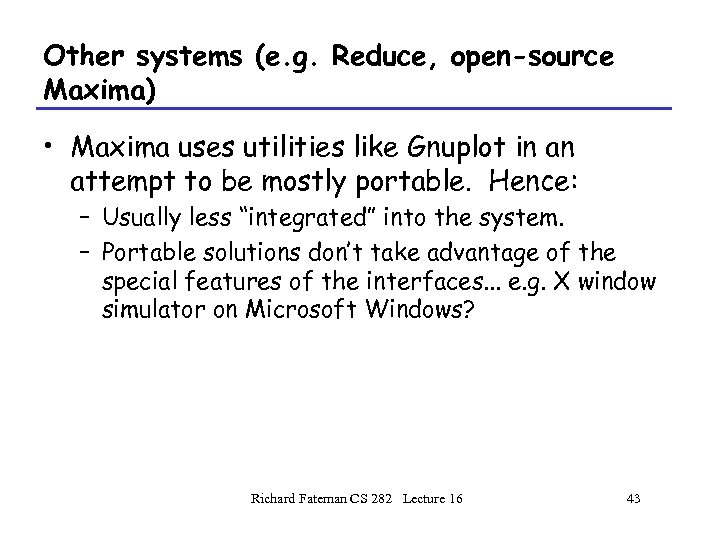Other systems (e. g. Reduce, open-source Maxima) • Maxima uses utilities like Gnuplot in an attempt to be mostly portable. Hence: – Usually less “integrated” into the system. – Portable solutions don’t take advantage of the special features of the interfaces. . . e. g. X window simulator on Microsoft Windows? Richard Fateman CS 282 Lecture 16 43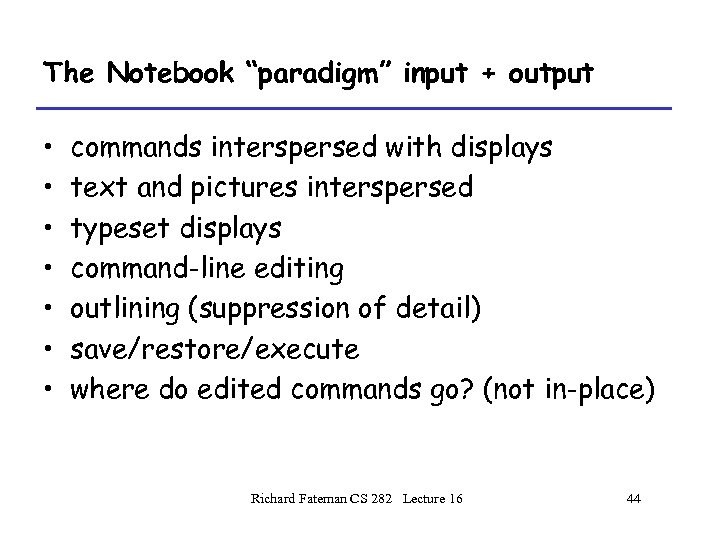The Notebook “paradigm” input + output • • commands interspersed with displays text and pictures interspersed typeset displays command-line editing outlining (suppression of detail) save/restore/execute where do edited commands go? (not in-place) Richard Fateman CS 282 Lecture 16 44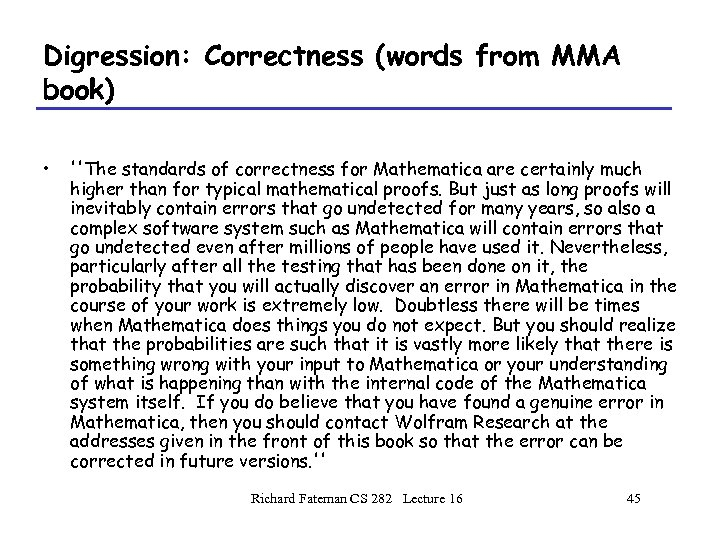Digression: Correctness (words from MMA book) • ''The standards of correctness for Mathematica are certainly much higher than for typical mathematical proofs. But just as long proofs will inevitably contain errors that go undetected for many years, so also a complex software system such as Mathematica will contain errors that go undetected even after millions of people have used it. Nevertheless, particularly after all the testing that has been done on it, the probability that you will actually discover an error in Mathematica in the course of your work is extremely low. Doubtless there will be times when Mathematica does things you do not expect. But you should realize that the probabilities are such that it is vastly more likely that there is something wrong with your input to Mathematica or your understanding of what is happening than with the internal code of the Mathematica system itself. If you do believe that you have found a genuine error in Mathematica, then you should contact Wolfram Research at the addresses given in the front of this book so that the error can be corrected in future versions. '' Richard Fateman CS 282 Lecture 16 45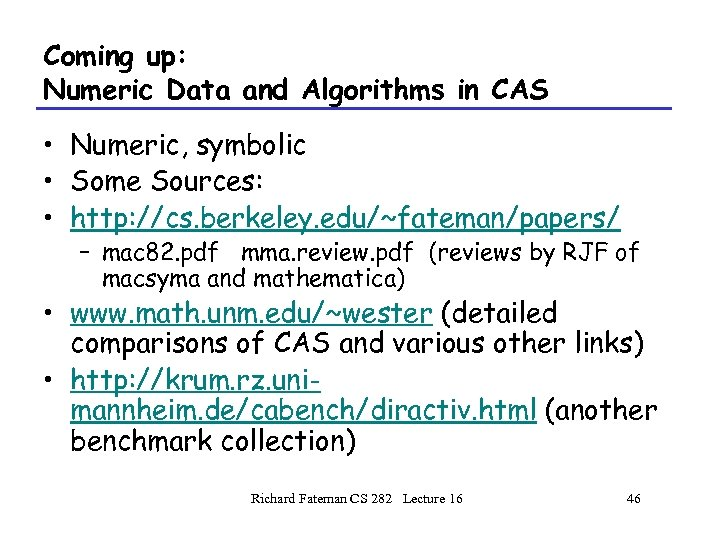Coming up: Numeric Data and Algorithms in CAS • Numeric, symbolic • Some Sources: • http: //cs. berkeley. edu/~fateman/papers/ – mac 82. pdf mma. review. pdf (reviews by RJF of macsyma and mathematica) • www. math. unm. edu/~wester (detailed comparisons of CAS and various other links) • http: //krum. rz. unimannheim. de/cabench/diractiv. html (another benchmark collection) Richard Fateman CS 282 Lecture 16 46